# Image Undistortion

This example shows how to remove lens distortion in images. The algorithm shown here is suitable for FPGAs.

Lens distortions are optical aberrations that deform images. Images typically have two main types of lens distortion: radial or tangential.

Radial distortion occurs when light rays bend more near the edges of a lens than they do at its optical center.Tangential distortion occurs when the lens and the image plane are not parallel.An undistort algorithm maps the coordinates of the output undistorted image to the input camera image by using distortion coefficients. The hardware-friendly undistort implementation in this example performs the same operation as the imrotate (Image Processing Toolbox) function.

As inputs to the undistort algorithm, you specify the intrinsic matrix and distortion coefficients that describe the image distortion to be corrected. The intrinsic matrix comprises the focal length, the optical center (also known as the principal point), and the skew coefficient. The distortion coefficients model radial and tangential distortions mathematically.

The computeCameraParameters function, included with this example, calculates the input parameters from the specified output image dimensions and a cameraParameters (Computer Vision Toolbox) object that describes the camera intrinsic matrix, distortion coefficients, and camera focal lengths in x- and y-directions. The cameraParameters object is provided in the cameraParams.mat file. This function also returns the displacement and offset parameters, which determine how much memory the undistort operation requires.

The example model calls the computeCameraParameters function in the PostLoadFcn callback, and then the model removes radial and tangential lens distortions in the input image by using the calculated parameters.

After undistortion, the hardware algorithm calculates the output pixel intensities by using bilinear interpolation. The implementation in this example does not require external DDR memory and instead stores and resamples the output pixel intensities by using on-chip block RAM.

### Image Undistortion Algorithm

The image undistortion algorithm maps the pixel locations of the output undistorted image to the pixels in the input distorted image by using a reverse mapping technique. This diagram shows the stages of the algorithm.Compute Camera Calibration Parameters

Camera calibration estimates the parameters of a lens and image sensor of an image or video camera. These parameters can be used to correct lens distortion, measure the size of an object in world units, or determine the location of the camera in the scene. Applications such as machine vision, robotic navigation systems, and 3-D scene reconstruction use these operations to detect and measure objects. Camera parameters include intrinsics, extrinsics, and distortion coefficients. This stage computes these parameters from a given input cameraParameters object and feeds them to the undistortion stage.

Undistortion

This stage removes distortion by using the distortion coefficients and the intrinsic matrix. These equations model distortion removal.

Let (u,v) be the coordinates of the input camera image and (x,y) be the undistorted pixel locations. Normalize x and y from pixel coordinates by translating to the optical center and dividing by the focal length in pixels.,,,,

where.,are radial distortion coefficients, and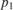,are tangential distortion coefficients. Then, calculate the final coeffient values by combining the radial and tangential components.Undistortion defines a mapping between the coordinates of the output undistorted image (u,v) and the coordinates of the distorted input camera image, (x,y).

Bilinear Interpolation

The image undistortion algorithm can produce noninteger values of (u,v). Generating the pixel intensity at each integer position requires a resampling technique such as interpolation. This example resamples the image intensity values corresponding to the generated coordinates by using bilinear interpolation.

In the equation and the diagram, (u,v) is the coordinate of the input pixel generated by the undistortion stage.,,, andare the four neighboring pixels, andand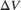are the displacements of the target pixel from its neighboring pixels. This stage of the algorithm computes the weighted average of the four neighboring pixels by using this equation.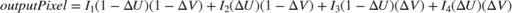### HDL Implementation

This figure shows the top-level view of the ImageUndistortHDL model. The InputImage block imports the image from a file. The Frame To Pixels block converts the input image frames to a pixel stream and a pixelcontrol bus for input to the ImageUndistortHDLAlgorithm subsystem. This subsystem removes distortions from the input image by using the distortion coefficients that you specify in the mask parameters. The Pixels To Frame block converts the stream of output pixels to a frame. The ImageViewer subsystem displays the input frame and the corresponding undistorted output.

open_system('ImageUndistortHDL'); set(allchild(0),'Visible','off');The PostLoadFcn callback of the example model imports the camera calibration parameters from the cameraParams.mat data file and computes the calibration parameters by calling the ComputeCameraParameters function provided with this example. Alternatively, you can generate your own camera calibration parameters and provide them as mask parameters of the ImageUndistortHDLAlgorithm subsystem.

In the ImageUndistortHDLAlgorithm subsystem, the GenerateControl subsystem uses the displacement parameter to modify the pixelcontrol bus from the input ctrl port. The CoordinateGeneration subsystem generates the row and column pixel coordinates (x,y) of the output undistorted image by using two HDL counters. The Undistortion subsystem maps the (x,y) position to its corresponding (u,v) position of the input camera image by using the distortion coefficients and camera intrinsics.

The AddressGeneration subsystem calculates the addresses of the four neighbors of (u,v) required for interpolation. This subsystem also computes the parameters,,, and, required for bilinear interpolation.

The Interpolation subsystem stores the pixel intensities of the input image in a memory modeled with a Simple Dual Port RAM block. To calculate each output pixel intensity, the subsystem reads the four neighbor pixel values and computes their weighted sum.

open_system('ImageUndistortHDL/ImageUndistortHDLAlgorithm','force');### Undistortion

The HDL implementation of undistortion takes the 3-by-3 camera intrinsic matrix, distortion coefficients, and the reciprocal ofandas masked parameters. The ComputeCameraParameters function, which is called in the PostLoadFcn callback of the model, generates these parameters. The intrinsic matrix is:The Undistortion subsystem implements the equations mentioned in the Image Undistortion Algorithm section by using Sum, Product, and Shift arithmetic blocks. The word length grows with each operation, and then the Denormalization subsystem truncates the word length to a size that ensures the precision and accuracy of the generated coordinates.

open_system('ImageUndistortHDL/ImageUndistortHDLAlgorithm/Undistortion','force');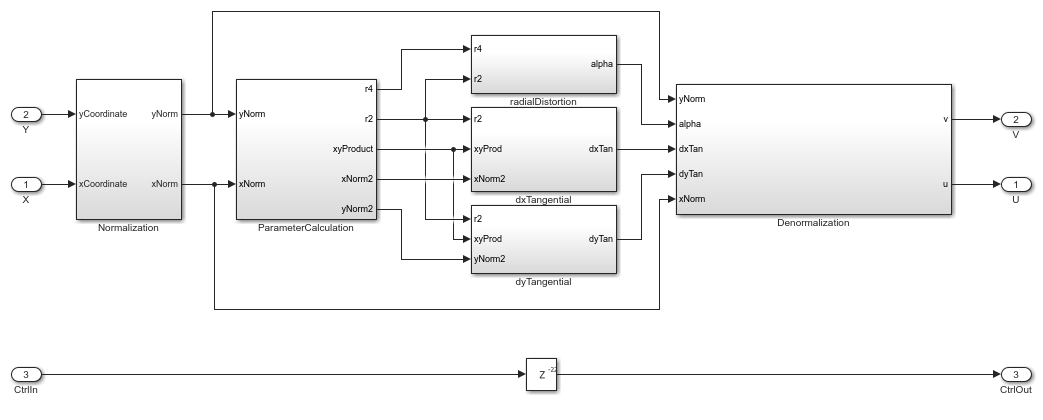The AddressGeneration subsystem calculates the displacementandof each pixel from its neighboring pixels by using the mapped coordinate (u,v) of the input raw image. The subsystem also rounds the coordinates to the nearest integer toward negative infinity.

open_system('ImageUndistortHDL/ImageUndistortHDLAlgorithm/AddressGeneration','force');The AddressCalculation subsystem checks the coordinates against the bounds of the input images. If any coordinate is outside the image dimensions, the subsystem sets the coordinate to the boundary value. Next, the subsystem calculates the index of the address for each of the four neighborhood pixels in the CacheMemory. The index represents the column of the cache. The subsystem finds the index for each address by using the even and odd nature of the incoming column and row coordinates, as determined by the Extract Bits block.

% ========================== % |Row || Col || Index || % ========================== % |Odd || Odd || 1 || % |Even || Odd || 2 || % |Odd || Even || 3 || % |Even || Even || 4 || % ========================== 

This equation specifies the address of the neighborhood pixels.is the row coordinate andis the column coordinate. When row is even, then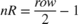. When row is odd, then. When col is even, then. When col is odd, then.

The IndexChangeForMemoryAccess MATLAB Function block in the AddressCalculation subsystem rearranges the addresses in increasing order of their indices. This operation ensures the correct fetching of data from the CacheMemory block. This subsystem passes the addresses to the CacheMemory subsystem, and passes Index,, andto the Interpolation subsystem.

The OutOfBound subsystem checks whether the (u,v) coordinates are out of bounds (that is, if any coordinate is outside the image dimensions). If the coordinate is out of bounds, the subsystem sets the corresponding output pixel to an intensity value of 0.

Finally, a Vector Concatenate block creates vectors of the addresses and indices.

### Interpolation

The Interpolation subsystem is a For Each block, which replicates its operation depending on the dimensions of the input pixel. For example, if the input is an RGB image, then the input pixel dimensions are 1-by-3, and the model includes three instances of this operation. Because the model uses a For Each block, it supports RGB or grayscale input. The operation inside the Interpolation subsystem comprises two subsystems: BilinearInterpolation and CacheMemory.

open_system('ImageUndistortHDL/ImageUndistortHDLAlgorithm/Interpolation','force');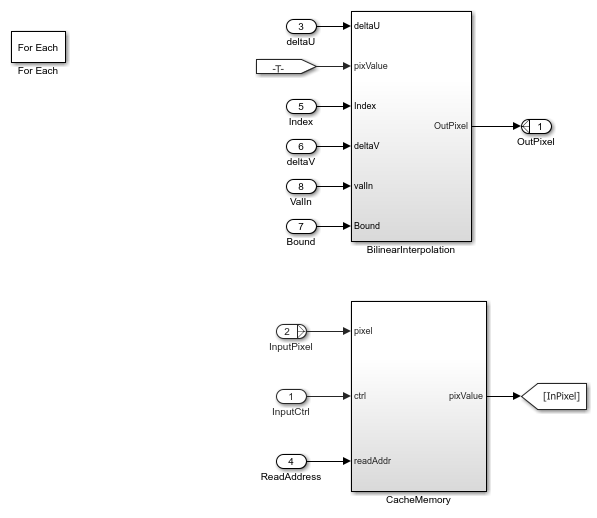Cache Memory

The CacheMemory subsystem contains a Simple Dual Port RAM block. The subsystem buffers the input pixels to form [Line 1 Pixel 1 | Line 2 Pixel 1 | Line 1 Pixel 2 | Line 2 Pixel 2] in the RAM. By using this configuration, the algorithm can read all four neighboring pixels in one cycle. The example calculates the required size of the cache memory from the offset output of the ComputeCameraParams function. The offset is the sum of the maximum deviation and the first row map. The first row map is the maximum value of the input image row coordinate that corresponds to the first row of the output undistorted image. The maximum deviation is the greatest difference between the maximum and minimum row coordinates for each row of the input image row map.

The WriteControl subsystem forms vectors of incoming pixels, write enables, and write addresses. The AddressGeneration subsystem provides a vector of read addresses. The vector of pixels from the RAM is the input to the BilinearInterpolation subsystem.

open_system('ImageUndistortHDL/ImageUndistortHDLAlgorithm/Interpolation/CacheMemory','force');Bilinear Interpolation

The BilinearInterpolation subsystem rearranges the vector of read pixels from the cache to their original indices. Then, the BilinearInterpolationEquation subsystem calculates a weighted sum of the neighborhood pixels by using the bilinear interpolation equation in the Image Undistortion Algorithm section. The result of the interpolation is the value of the output undistorted pixel.

open_system('ImageUndistortHDL/ImageUndistortHDLAlgorithm/Interpolation/BilinearInterpolation','force');### Simulation and Results

This example uses a 510-by-510 grayscale input image. The input pixels use the uint8 data type for either grayscale or RGB input images.

This figure shows the input distorted image and the corresponding output undistorted image for the camera parameters provided in cameraParams.mat. The results of the ImageUndistortHDL model for this input matches the output of the undistortImage function.To check and generate the HDL code referenced in this example, you must have the HDL Coder™ product.

To generate the HDL code, use this command.

makehdl('ImageUndistortHDL/ImageUndistortHDLAlgorithm') 

To generate the test bench, use this command.

makehdltb('ImageUndistortHDL/ImageUndistortHDLAlgorithm') 

This design was synthesized using Xilinx® Vivado® for the Xilinx (R) Zynq® ZC706 device and met a timing requirement of over 200 MHz. This table shows the resource utilization for the HDL subsystem.

% =============================================================== % |Model Name || ImageUndistortHDL || % =============================================================== % |Input Image Resolution || 510 x 510 || % |LUT || 2806 || % |FF || 2832 || % |BRAM || 17 || % |Total DSP Blocks || 53 || % ===============================================================